23:50

# Learn Basic Data Analysis with SQL Window Functions

There are many alternatives to T-SQL when it comes to data analysis. However, when improvements over time and introduction of Window functions are considered, T-SQL is capable of performing data analysis on a basic level and, in some cases, even beyond that.

First, let’s first get familiar with SQL Window functions in the context of the Microsoft documentation.

### Microsoft Definition

A window function computes a value for each row in the window.

### Simple Definition

A window function helps us to focus on a particular portion (window) of the result set so that we can perform data analysis on that specific part (window) only, rather than on the entire result set.

In other words, SQL window functions turn a result set into several smaller sets for data analysis purposes.

### What is a result set

Simply put, a result set consists of all records retrieved by running a SQL query.

For example, we can create a table named Product and insert the following data into it:

```-- (1) Create the Product table
CREATE TABLE [dbo].[Product]
(
[ProductId] INT NOT NULL PRIMARY KEY,
[Name] VARCHAR(40) NOT NULL,
[Region] VARCHAR(40) NOT NULL
)

-- (2) Populate the Product table
INSERT INTO Product
(ProductId,Name,Region)
VALUES

Now, the result set retrieved by using the script below will contain all rows from the Product table:

```-- (3) Result set
SELECT [ProductId], [Name],[Region] FROM Product```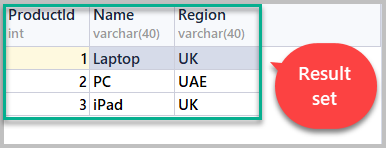### What is a Window

It is important to first understand the concept of a window as it relates to SQL window functions. In this context, a window is just a way of narrowing down your scope by targeting a specific part of the result set (as we already mentioned above).

You may be wondering now – what does ‘targeting a specific part of the result set’ actually means?

Returning to the example we looked at, we can create a SQL window based on the product region by dividing the result set into two windows.

### Understanding Row_Number()

To proceed, we will need to use the Row_Number() function which temporarily gives a sequence number to the output rows.

For example, if we want to add a row numbers to the result set based on ProductID, we’ll need to use ROW_NUMBER() to order it by Product ID as follows:

```--Using the row_number() function to order the result set by ProductID
SELECT ProductID,ROW_NUMBER() OVER (ORDER BY ProductID) AS SrNo,Name,Region FROM Product```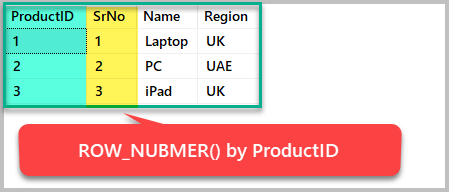Now, if we want the Row_Number() function to order the result set by ProductID descending, then the sequence of output rows based on ProductID will change as follows:

```--Using the row_number() function to order the result set by ProductID descending
SELECT ProductID,ROW_NUMBER() OVER (ORDER BY ProductID DESC) AS SrNo,Name,Region FROM Product```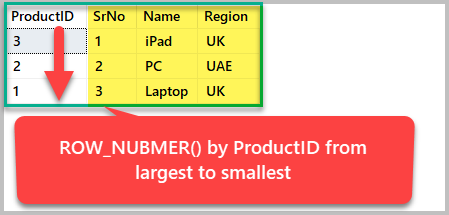There are no SQL windows yet since the only thing we’ve done is ordering the set by specific criteria. As discussed earlier, windowing means breaking the result set into several smaller sets to analyze each one of them separately.

### Creating a Window with Row_Number()

To create a SQL window in our result set, we will need to to partition it based on any of the columns it contains.

We can now partition the result set by region as follows:

```--Creating a SQL window based on Region
SELECT ROW_NUMBER() OVER (Partition by region ORDER BY Region) as Region_Serial_Number
, Name, Region FROM dbo.Product```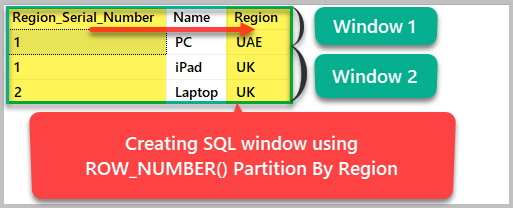### Select – Over Clause

In other words, Select with the Over clause paves the way for SQL window functions by partitioning a result set into smaller windows.

According to the Microsoft documentation, Select with the Over clause defines a window which can then be used by any window function.

Now, let’s create a table called KitchenProduct as follows:

```CREATE TABLE [dbo].[KitchenProduct]
(
[KitchenProductId] INT NOT NULL PRIMARY KEY IDENTITY(1,1),
[Name] VARCHAR(40) NOT NULL,
[Country] VARCHAR(40) NOT NULL,
[Quantity] INT NOT NULL,
[Price] DECIMAL(10,2) NOT NULL
);
GO

INSERT INTO dbo.KitchenProduct
(Name, Country, Quantity, Price)
VALUES
('Kettle','Germany',10,15.00)
,('Kettle','UK',20,12.00)
,('Toaster', 'France',10,10.00)
,('Toaster','UAE',10,12.00)
,('Kitchen Clock','UK',50,20.00)
,('Kitchen Clock','UAE',35,15.00)```

Now, let’s view the table:

`SELECT [KitchenProductId], [Name], [Country], [Quantity], [Price] FROM dbo.KitchenProduct`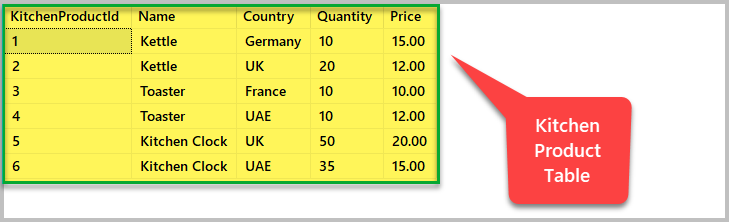If you want to see each product with its own serial number rather than a number based on the generalized product ID, then you would have to use a SQL window function to partition the result set by product as follows:

```-- Viewing each product in its own series
SELECT ROW_NUMBER() OVER (Partition by Name order by Name) Product_SrNo,Name,Country,Quantity
FROM dbo.KitchenProduct```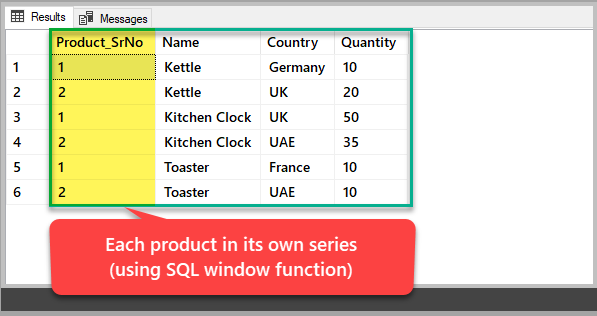### Compatibility (Select – Over Clause)

According to Microsoft documentation, Select – Over Clause is compatible with the following SQL database versions:

1. SQL Server 2008 and upward
2. Azure SQL Database
3. Azure SQL Data Warehouse
4. Parallel Data Warehouse

### Syntax

SELECT – OVER (Partition by <column> Order by <column>)

Please note that I have simplified the syntax to make it easy to understand; please refer to the Microsoft documentation to see the full syntax.

## Pre-requisites

### Familiarity with T-SQL

This article assumes that the readers have a basic knowledge of T-SQL and are capable of writing and running basic SQL scripts.

### Setup the Sales sample table

This article requires the following sample table so that we can run our SQL window function examples:

```-- (1) Create the Sales sample table
CREATE TABLE [dbo].[Sales]
(
[SalesId] INT NOT NULL IDENTITY(1,1),
[Product] VARCHAR(40) NOT NULL,
[Date] DATETIME2,
[Revenue] DECIMAL(10,2),
CONSTRAINT [PK_Sales] PRIMARY KEY ([SalesId])
);
GO

-- (2) Populating the Sales sample table
SET IDENTITY_INSERT [dbo].[Sales] ON
INSERT INTO [dbo].[Sales] ([SalesId], [Product], [Date], [Revenue]) VALUES (1, N'Laptop', N'2017-01-01 00:00:00', CAST(200.00 AS Decimal(10, 2)))
INSERT INTO [dbo].[Sales] ([SalesId], [Product], [Date], [Revenue]) VALUES (2, N'PC', N'2017-01-01 00:00:00', CAST(100.00 AS Decimal(10, 2)))
INSERT INTO [dbo].[Sales] ([SalesId], [Product], [Date], [Revenue]) VALUES (3, N'Mobile Phone', N'2018-01-01 00:00:00', CAST(300.00 AS Decimal(10, 2)))
INSERT INTO [dbo].[Sales] ([SalesId], [Product], [Date], [Revenue]) VALUES (4, N'Accessories', N'2018-01-01 00:00:00', CAST(150.00 AS Decimal(10, 2)))
INSERT INTO [dbo].[Sales] ([SalesId], [Product], [Date], [Revenue]) VALUES (5, N'iPad', N'2019-01-01 00:00:00', CAST(300.00 AS Decimal(10, 2)))
INSERT INTO [dbo].[Sales] ([SalesId], [Product], [Date], [Revenue]) VALUES (6, N'PC', N'2019-01-01 00:00:00', CAST(200.00 AS Decimal(10, 2)))
INSERT INTO [dbo].[Sales] ([SalesId], [Product], [Date], [Revenue]) VALUES (7, N'Laptop', N'2019-01-01 00:00:00', CAST(300.00 AS Decimal(10, 2)))
SET IDENTITY_INSERT [dbo].[Sales] OFF```

View all sales by running the following script:

```-- View sales
SELECT
[SalesId],[Product],[Date],[Revenue]
FROM dbo.Sales```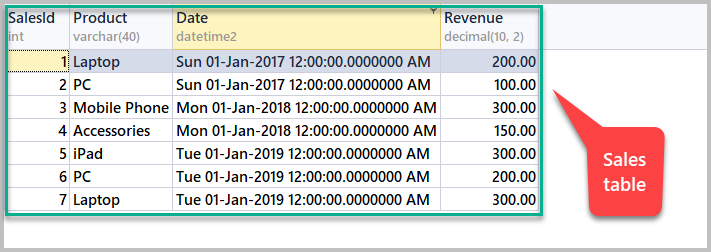## Group By vs SQL Window Functions

One can wonder – what is the difference between using the Group By clause and SQL window functions?

Well, the answer lies in the examples below.

### Group By Example

In order to see total sales by product, we can use Group By as follows:

```-- Total sales by product using Group By
SELECT
Product
,SUM(REVENUE) AS Total_Sales
FROM dbo.Sales
GROUP BY Product
ORDER BY Product```So, the Group By clause helps us see the total sales. The total sales value is the sum of revenue for all similar products in the same row with no Group By clause used. What if we are interested in seeing the revenue (sale) of each individual product along with total sales?

This is where SQL window functions come into action.

### SQL Window Function Example

In order to see the product, revenue and total revenue by all similar products, we have to partition the data on a by-product basis using OVER() as follows:

```-- Total sales by product using an SQL window function
SELECT
Product
,REVENUE
,SUM(REVENUE) OVER (PARTITION BY PRODUCT) AS Total_Sales
FROM dbo.Sales```

The output should be as follows: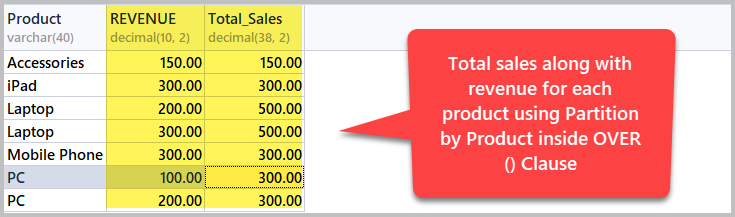So, we can now easily see the sales for each individual product along with total sales for that product. For example, the revenue for PC is 100.00 but total sales (sum of revenue for the PC product) is 300.00 because two different PC models were being sold.

### Basic Analysis with the Aggregate Functions

Aggregate functions return a single value after performing calculations on a set of data.

In this section, we are going to further explore SQL window functions – specifically, by using them along with aggregate functions to perform basic data analysis.

### Common Aggregate Functions

The most common aggregate functions are:

1. Sum
2. Count
3. Min
4. Max
5. Avg (Average)

#### Aggregate Data Analysis by Product

In order to analyse the result set on a by-product basis with the help of aggregate functions, we simply have to use an aggregate function with a by-product partition inside of the OVER() statement:

```-- Data analysis by product using aggregate functions
SELECT Product,Revenue
,SUM(REVENUE) OVER (PARTITION BY PRODUCT) as Total_Sales
,MIN(REVENUE) OVER (PARTITION BY PRODUCT) as Minimum_Sales
,MAX(REVENUE) OVER (PARTITION BY PRODUCT) as Maximum_Sales
,AVG(REVENUE) OVER (PARTITION BY PRODUCT) as Average_Sales
FROM dbo.Sales```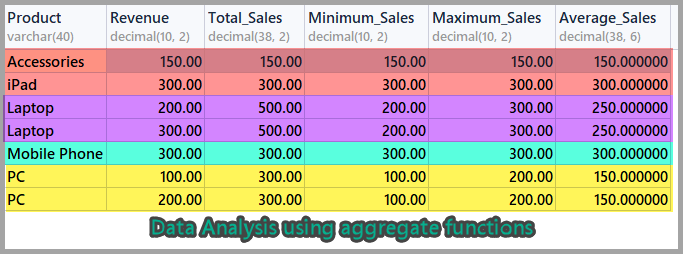If you take a closer look at the PC or Laptop products, you will see how aggregate functions are working together alongside the SQL window function.

In the example above, we can see that the Revenue value for PC is 100.00 first time and 200.00 next time, but Total Sales amount to 300.00. The similar information can be seen for the rest of the aggregate functions.

#### Aggregate Data Analysis by Date

Now, let’s perform some data analysis of the products on a by-date basis using SQL window functions in combination with aggregate functions.

This time, we are going to partition the result set by date rather than by product as follows:

```-- Data analysis by date using aggregate functions
SELECT Product,date,Revenue
,SUM(REVENUE) OVER (PARTITION BY DATE) as Total_Sales
,MIN(REVENUE) OVER (PARTITION BY DATE) as Minimum_Sales
,MAX(REVENUE) OVER (PARTITION BY DATE) as Maximum_Sales
,AVG(REVENUE) OVER (PARTITION BY DATE) as Average_Sales
FROM dbo.Sales```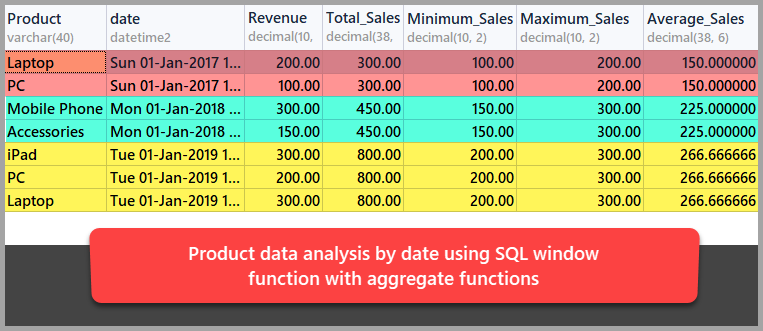With this, we have learned basic data analysis techniques using the SQL window functions approach.

## Things to do

Now that you are familiar with SQL window functions, please try the following:

1. Keeping in mind the examples we looked at, perform basic data analysis using SQL window functions on the sample database mentioned in this article.
2. Adding a Customer column to the Sales sample table and see how rich your data analysis can become when another column (customer) is added to it.
3. Adding a Region column to the Sales sample table and perform basic data analysis using aggregate functions by region.